# Maths - Euler to Matrix - Sample Orientations

## Sample Rotations

In order to try to explain things and give some examples we can try I thought it might help to show the rotations for a finite subset of the rotation group. We will use the set of rotations of a cube onto itself, this is a permutation group which gives 24 possible rotations as explaned on this page.

heading applied first giving 4 possible orientations:reference orientation

attitude = 0
bank = 0

 ch*ca -ch*sa*cb + sh*sb ch*sa*sb + sh*cb sa ca*cb -ca*sb -sh*ca sh*sa*cb + ch*sb - sh*sa*sb + ch*cb

ch=1
ca=1
cb=1
sh=0
sa=0
sb=0

 1 0 0 0 1 0 0 0 1rotate by 90 degrees about y axis

attitude = 0
bank = 0

 ch*ca -ch*sa*cb + sh*sb ch*sa*sb + sh*cb sa ca*cb -ca*sb -sh*ca sh*sa*cb + ch*sb - sh*sa*sb + ch*cb

ch=0
ca=1
cb=1
sh=1
sa=0
sb=0

 0 0 1 0 1 0 -1 0 0rotate by 180 degrees about y axis

attitude = 0
bank = 0

 ch*ca -ch*sa*cb + sh*sb ch*sa*sb + sh*cb sa ca*cb -ca*sb -sh*ca sh*sa*cb + ch*sb - sh*sa*sb + ch*cb

ch=-1
ca=1
cb=1
sh=0
sa=0
sb=0

 -1 0 0 0 1 0 0 0 -1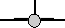rotate by 270 degrees about y axis

attitude = 0
bank = 0

 ch*ca -ch*sa*cb + sh*sb ch*sa*sb + sh*cb sa ca*cb -ca*sb -sh*ca sh*sa*cb + ch*sb - sh*sa*sb + ch*cb

ch=0
ca=1
cb=1
sh=-1
sa=0
sb=0

 0 0 -1 0 1 0 1 0 0

Then apply attitude +90 degrees for each of the above: (note: that if we went on to apply bank to these it would just rotate between these values, the straight up and streight down orientations are known as singularities because they can be fully defined without using the bank value)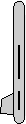attitude = 90 degrees
bank = 0

 ch*ca -ch*sa*cb + sh*sb ch*sa*sb + sh*cb sa ca*cb -ca*sb -sh*ca sh*sa*cb + ch*sb - sh*sa*sb + ch*cb

ch=1
ca=0
cb=1
sh=0
sa=1
sb=0

 0 -1 0 1 0 0 0 0 1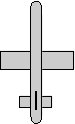attitude = 90 degrees
bank = 0

 ch*ca -ch*sa*cb + sh*sb ch*sa*sb + sh*cb sa ca*cb -ca*sb -sh*ca sh*sa*cb + ch*sb - sh*sa*sb + ch*cb

ch=0
ca=0
cb=1
sh=1
sa=1
sb=0

 0 0 1 1 0 0 0 1 0attitude = 90 degrees
bank = 0

 ch*ca -ch*sa*cb + sh*sb ch*sa*sb + sh*cb sa ca*cb -ca*sb -sh*ca sh*sa*cb + ch*sb - sh*sa*sb + ch*cb

ch=-1
ca=0
cb=1
sh=0
sa=1
sb=0

 0 1 0 1 0 0 0 0 -1attitude = 90 degrees
bank = 0

 ch*ca -ch*sa*cb + sh*sb ch*sa*sb + sh*cb sa ca*cb -ca*sb -sh*ca sh*sa*cb + ch*sb - sh*sa*sb + ch*cb

ch=0
ca=0
cb=1
sh=-1
sa=1
sb=0

 0 0 -1 1 0 0 0 -1 0

Or instead apply attitude -90 degrees (also a singularity):attitude = -90 degrees
bank = 0

 ch*ca -ch*sa*cb + sh*sb ch*sa*sb + sh*cb sa ca*cb -ca*sb -sh*ca sh*sa*cb + ch*sb - sh*sa*sb + ch*cb

ch=1
ca=0
cb=1
sh=0
sa=-1
sb=0

 0 1 0 -1 0 0 0 0 1attitude = -90 degrees
bank = 0

 ch*ca -ch*sa*cb + sh*sb ch*sa*sb + sh*cb sa ca*cb -ca*sb -sh*ca sh*sa*cb + ch*sb - sh*sa*sb + ch*cb

ch=0
ca=0
cb=1
sh=1
sa=-1
sb=0

 0 0 1 -1 0 0 0 -1 0attitude = -90 degrees
bank = 0

 ch*ca -ch*sa*cb + sh*sb ch*sa*sb + sh*cb sa ca*cb -ca*sb -sh*ca sh*sa*cb + ch*sb - sh*sa*sb + ch*cb

ch=-1
ca=0
cb=1
sh=0
sa=-1
sb=0

 0 -1 0 -1 0 0 0 0 -1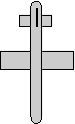attitude = -90 degrees
bank = 0

 ch*ca -ch*sa*cb + sh*sb ch*sa*sb + sh*cb sa ca*cb -ca*sb -sh*ca sh*sa*cb + ch*sb - sh*sa*sb + ch*cb

ch=0
ca=0
cb=1
sh=-1
sa=-1
sb=0

 0 0 -1 -1 0 0 0 1 0

Normally we dont go beond attitude + or - 90 degrees because thes are singularities, instead apply bank +90 degrees: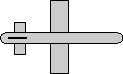attitude = 0
bank = 90 degrees

 ch*ca -ch*sa*cb + sh*sb ch*sa*sb + sh*cb sa ca*cb -ca*sb -sh*ca sh*sa*cb + ch*sb - sh*sa*sb + ch*cb

ch=1
ca=1
cb=0
sh=0
sa=0
sb=1

 1 0 0 0 0 -1 0 1 0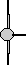attitude = 0
bank = 90 degrees

 ch*ca -ch*sa*cb + sh*sb ch*sa*sb + sh*cb sa ca*cb -ca*sb -sh*ca sh*sa*cb + ch*sb - sh*sa*sb + ch*cb

ch=0
ca=1
cb=0
sh=1
sa=0
sb=1

 0 1 0 0 0 -1 -1 0 0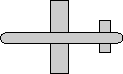attitude = 0
bank = 90 degrees

 ch*ca -ch*sa*cb + sh*sb ch*sa*sb + sh*cb sa ca*cb -ca*sb -sh*ca sh*sa*cb + ch*sb - sh*sa*sb + ch*cb

ch=-1
ca=1
cb=0
sh=0
sa=0
sb=1

 -1 0 0 0 0 -1 0 -1 0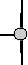attitude = 0
bank = 90 degrees

 ch*ca -ch*sa*cb + sh*sb ch*sa*sb + sh*cb sa ca*cb -ca*sb -sh*ca sh*sa*cb + ch*sb - sh*sa*sb + ch*cb

ch=0
ca=1
cb=0
sh=-1
sa=0
sb=1

 0 -1 0 0 0 -1 1 0 0

Apply bank +180 degrees:attitude = 0
bank = 180 degrees

 ch*ca -ch*sa*cb + sh*sb ch*sa*sb + sh*cb sa ca*cb -ca*sb -sh*ca sh*sa*cb + ch*sb - sh*sa*sb + ch*cb

ch=1
ca=1
cb=-1
sh=0
sa=0
sb=0

 1 0 0 0 -1 0 0 0 -1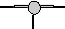attitude = 0
bank = 180 degrees

 ch*ca -ch*sa*cb + sh*sb ch*sa*sb + sh*cb sa ca*cb -ca*sb -sh*ca sh*sa*cb + ch*sb - sh*sa*sb + ch*cb

ch=0
ca=1
cb=-1
sh=1
sa=0
sb=0

 0 0 -1 0 -1 0 -1 0 0attitude = 0
bank = 180 degrees

 ch*ca -ch*sa*cb + sh*sb ch*sa*sb + sh*cb sa ca*cb -ca*sb -sh*ca sh*sa*cb + ch*sb - sh*sa*sb + ch*cb

ch=-1
ca=1
cb=-1
sh=0
sa=0
sb=0

 -1 0 0 0 -1 0 0 0 1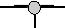attitude = 0
bank = 180 degrees

 ch*ca -ch*sa*cb + sh*sb ch*sa*sb + sh*cb sa ca*cb -ca*sb -sh*ca sh*sa*cb + ch*sb - sh*sa*sb + ch*cb

ch=0
ca=1
cb=-1
sh=-1
sa=0
sb=0

 0 0 1 0 -1 0 1 0 0

Apply bank -90 degrees: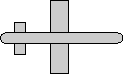attitude = 0
bank = -90 degrees

 ch*ca -ch*sa*cb + sh*sb ch*sa*sb + sh*cb sa ca*cb -ca*sb -sh*ca sh*sa*cb + ch*sb - sh*sa*sb + ch*cb

ch=1
ca=1
cb=0
sh=0
sa=0
sb=-1

 1 0 0 0 0 1 0 -1 0attitude = 0
bank = -90 degrees

 ch*ca -ch*sa*cb + sh*sb ch*sa*sb + sh*cb sa ca*cb -ca*sb -sh*ca sh*sa*cb + ch*sb - sh*sa*sb + ch*cb

ch=0
ca=1
cb=0
sh=1
sa=0
sb=-1

 0 -1 0 0 0 1 -1 0 0attitude = 0
bank = -90 degrees

 ch*ca -ch*sa*cb + sh*sb ch*sa*sb + sh*cb sa ca*cb -ca*sb -sh*ca sh*sa*cb + ch*sb - sh*sa*sb + ch*cb

ch=-1
ca=1
cb=0
sh=0
sa=0
sb=-1

 -1 0 0 0 0 1 0 1 0attitude = 0
bank = -90 degrees

 ch*ca -ch*sa*cb + sh*sb ch*sa*sb + sh*cb sa ca*cb -ca*sb -sh*ca sh*sa*cb + ch*sb - sh*sa*sb + ch*cb

ch=0
ca=1
cb=0
sh=-1
sa=0
sb=-1

 0 1 0 0 0 1 1 0 0